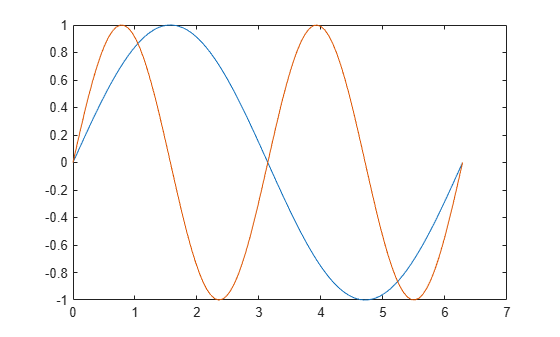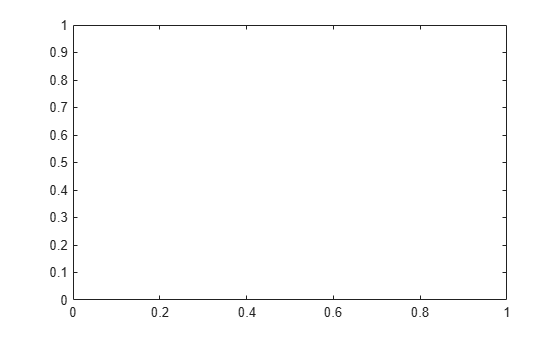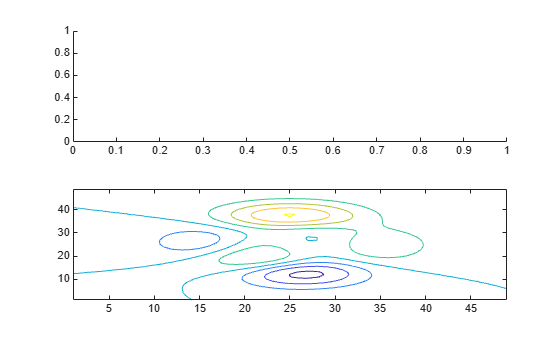# cla

## Syntax

``cla``
``cla(ax)``
``cla reset``
``cla(ax,'reset')``

## Description

example

````cla` deletes all graphics objects that have visible handles from the current axes. The handle is visible if the `HandleVisibility` property of the object is set to `'on'`. The next plot added to the axes uses the first color and line style based on the `ColorOrder` and `LineStyleOrder` properties of the axes. If axes do not exist, then this command creates one.```

example

````cla(ax)` deletes graphics objects from the axes, polar axes, or geographic axes specified by `ax` instead of the current axes.```

example

````cla reset` deletes graphics objects from the current axes regardless of their handle visibility. It also resets axes properties to their default values, except for the `Position` and `Units` properties.```

example

````cla(ax,'reset')` resets properties for the specified axes.```

## Examples

collapse all

Plot two sine waves. Then, clear the line plots from the axes.

```x = linspace(0,2*pi); y1 = sin(x); plot(x,y1) hold on y2 = sin(2*x); plot(x,y2)````cla``cla` clears the line plots and resets the `ColorIndex` and `LineStyleIndex` properties of the axes to 1. Subsequent plots start from the beginning of the color order and line style order. For example, plot another sine wave.

```y3 = sin(3*x); plot(x,y3) hold off```Starting in R2019b, you can display a tiling of plots using the `tiledlayout` and `nexttile` functions. Call the `tiledlayout` function to create a 2-by-1 tiled chart layout. Call the `nexttile` function to create the axes objects `ax1` and `ax2`. Add plots to both axes.

```tiledlayout(2,1) ax1 = nexttile; surf(ax1,peaks) ax2 = nexttile; contour(ax2,peaks)```Clear the surface plot from the upper axes by specifying `ax1` as an input argument to `cla`.

`cla(ax1)`Now, reset all properties for the axes, including the camera properties that control the view, by using the optional input argument `'reset'`.

`cla(ax1,'reset')`Create a line plot and set the axis limits.

```x = linspace(0,2*pi); y = sin(x); plot(x,y) axis([0 5 -2 2])```Clear the line plot from the axes and reset all the axes properties to their default values. `cla reset` resets all properties of the current axes, except for the `Position` and `Units` properties.

`cla reset`## Input Arguments

collapse all

Target axes, specified as an `Axes` object, a `PolarAxes` object, or a `GeographicAxes` object. Use `ax` to clear specific axes, instead of the current axes.

## Algorithms

The `cla` command resets the `ColorOrderIndex` and `LineStyleOrderIndex` properties of the current axes to `1`.